Next: Optimal Information Transmission Up: Associative Decorrelation Dynamics Previous: Dynamic Equations

## Dynamic Stability

It is straight forward to show that when the time constants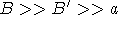, the dynamics reduces to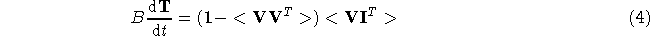where bold-faced quantities are matrices and vectors and <> denotes ensemble average. It is not difficult to show that this equation has a Lyapunov or ``energy'' function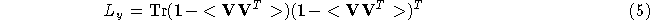which is lower bounded and satisfies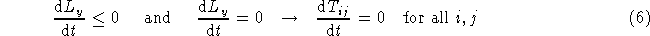Thus the dynamics is stable. When it is stable, the output activities are decorrelated,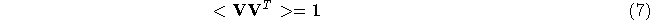The above equation shows that this dynamics always leads to a stable state where the neuronal activities are decorrelated and their correlation matrix is orthonormal. Yet the connections change in an associative fashion --- equation (3) and (3) are almost Hebbian. That is why we call it associative decorrelation dynamics. From information processing point of view, a network, self-organized to satisfy equation (7), is optimized for Gaussian input ensembles and white output noises, as shown next.Next: Optimal Information Transmission Up: Associative Decorrelation Dynamics Previous: Dynamic Equations